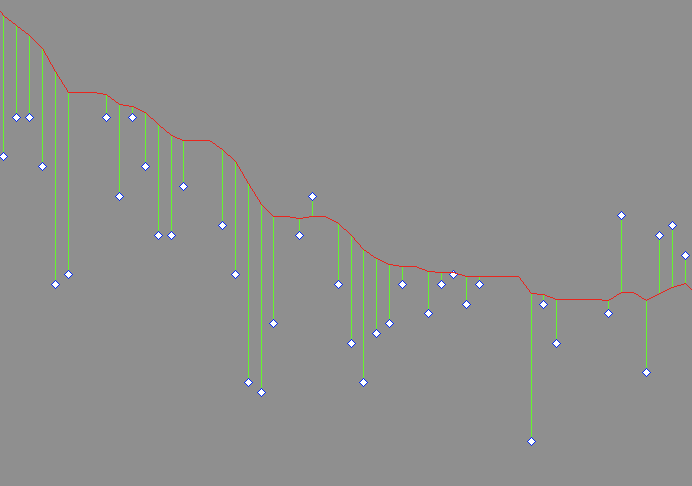# Can matplotlib generate charts like this?

Hi all,

Can matplotlib (or any other Python charting library) generate charts
like this: (also attached if you prefer)

http://imagebin.ca/view/iGhEQEE.html

It's basically a moving average with the vertical lines being the
difference between the average and the actual data point.

Can anyone send me in the right direction?

Thanks!

ErikErik Wickstrom schrieb:

Hi all,

Can matplotlib (or any other Python charting library) generate charts
like this: (also attached if you prefer)

Here is a demo-script. The second way is inspired by matlab. Can this be
done more easily in python? Can X and Y be built more elegantly with numpy?

Armin
-------8<------------------------
from pylab import *
# data generation
x = linspace(0,10,100)
y = exp(-x)
ye = y + rand(y.size)-0.5
# plot the vertical lines
# with loop
for xl,yl,yel in zip(x,y,ye):
plot([xl,xl],[yl,yel],'r')
plot(x,y,x,ye,'d')

# plot by separating with NaN
figure()
X = zeros((x.size,3))
Y = zeros((x.size,3))
X[:,0],X[:,1],X[:,2] = x,x,NaN
Y[:,0],Y[:,1],Y[:,2] = y,ye,NaN
plot(X.flatten(),Y.flatten(),x,y,x,ye,'d')

show()
-------8<------------------------

Erik Wickstrom wrote:

Hi all,

Can matplotlib (or any other Python charting library) generate charts
like this: (also attached if you prefer)

http://imagebin.ca/view/iGhEQEE.html

It's basically a moving average with the vertical lines being the
difference between the average and the actual data point.

Can anyone send me in the right direction?

Can you adapt one of the examples here:

http://matplotlib.sourceforge.net/examples/pylab_examples/errorbar_demo.html

Jason

Erik Wickstrom wrote:

Hi all,

Can matplotlib (or any other Python charting library) generate charts
like this: (also attached if you prefer)

http://imagebin.ca/view/iGhEQEE.html

It's basically a moving average with the vertical lines being the
difference between the average and the actual data point.

It looks like what you need is a simple modification of the present stem plot:

http://matplotlib.sourceforge.net/examples/pylab_examples/stem_plot.html

For now, you may be able to use the source--the stem method of the Axes class in matplotlib/lib/matplotlib/axes.py--to come up with your own function to do the job. Longer term, you, I, or someone else, should add this capability to that method via a keyword argument giving the baseline as a constant, or as an array of points corresponding to the input x variable. Even more options are possible.

Eric

···

Can anyone send me in the right direction?

Thanks!

Erik

------------------------------------------------------------------------

------------------------------------------------------------------------

------------------------------------------------------------------------------
Let Crystal Reports handle the reporting - Free Crystal Reports 2008 30-Day trial. Simplify your report design, integration and deployment - and focus on what you do best, core application coding. Discover what's new with Crystal Reports now. http://p.sf.net/sfu/bobj-july

------------------------------------------------------------------------

_______________________________________________
Matplotlib-users mailing list
Matplotlib-users@lists.sourceforge.net
https://lists.sourceforge.net/lists/listinfo/matplotlib-users